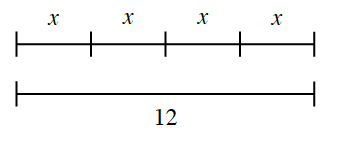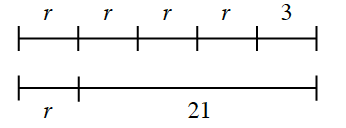### Home > CCAA > Chapter 4 Unit 3 > Lesson CC2: 4.2.2 > Problem4-43

4-43.

Each of the diagrams below represents a sequence for a tightrope walker. For each one, explain the sequence and find the length of the unknown trick.

1.Since the tightrope walker performs the trick $4$ times and goes a total of $12$ units, this can be thought of as what value times $4$ will give $12$?

Four times $3$ equals $12$.

$x=3$
The trick is $3$ units long.

1.The first time he crosses, the tightrope walker performs the trick $4$ times and then walks $3$ units. Remember to describe the second trip and to find the length of the unknown trick.